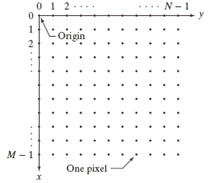# Digital image processing using MATLAB: digital image representation

Today I'm starting an regular, occasional series with tutorial material on digital image processing using MATLAB. I'm going to look at topics in roughly the order used in the book Digital Image Processing Using MATLAB, Gatesmark Publishing, 2009, by Gonzalez, Woods, and Eddins. (Yes, that's me. It was a lot of nights and weekends.)

### Digital image representation

Let's start with digital image representation. (I do not plan to cover image formation. For that topic, see Chapter 2 of Digital Image Processing, Prentice Hall, 2008, by Gonzalez and Woods.) The common mathematical representation of an image is a function of two continuous spatial coordinates: $f(x,y)$. The value $f(x_0,y_0)$ is often called the image intensity at $(x_0,y_0)$, although that term is used loosely because it often does not represent actual light intensity. The term gray level is also commonly used.

You can represent a color image mathematically in a couple of different ways. One way is to use a collection of image functions, one per color component, such as $r(x,y)$, $g(x,y)$, and $b(x,y)$. Another way is to use a vector-valued function, ${\mathbf f}(x,y)$.

Many functions in the Image Processing Toolbox also support higher-dimensional images. For example, $f$ might be a function of three spatial variable, as in $f(x,y,z)$. Image Processing Toolbox documentation calls this a multidimensional image.

Converting the amplitude values of $f$ to a set of discrete values is called quantization. Capturing those values at discrete spatial coordinates is called sampling. When $x$, $y$, and the values of $f$ are all finite, discrete quantities, we call f a digital image.

### Coordinate conventions

It's important to pay attention to different coordinate system conventions that may be used in image processing. Often the center of the upper-left pixel is considered to be the origin, (0,0). There is more variation in the assignment of $x$ and $y$ axes. The coordinate system in the diagram below, with the $x$-axis pointing down and the $y$-axis pointing to the right, is used in Digital Image Processing Using MATLAB in order to be consistent with the Gonzalez and Woods book, Digital Image Processing.From Figure 2.1, Digital Image Processing Using MATLAB, 2nd ed. Used with permission.

When displaying images in MATLAB, the usual convention is for the center of the upper-left pixel to be at (1,1), the $x$-axis to point to the right, and the $y$-axis to point down.

### Images as matrices and arrays

Digital images are very conveniently represented as matrices, which happens to be great for working with in MATLAB. A monochrome image matrix looks like this:

   f(1,1)  f(1,2)  ...  f(1,N)
f(2,1)  f(2,2)  ...  f(2,N)
.       .            .
.       .            .
.       .            .
f(M,1)  f(M,2)  ...  f(M,N)

(This happy marriage of a convenient digital image representation and the MATLAB strength at working with matrices is, more or less, the reason I ended up working at MathWorks.)

We represent color images in MATLAB as multidimensional arrays. For example, an RGB image (with three color components) is represented as an M-by-N-by-3 array. A CMYK image (with four color components) would use an M-by-N-by-4 array.

Multidimensional images are also represented in MATLAB as multidimensional arrays. Therefore we rely on context to distinguish between an RGB image (M-by-N-by-3) and a three-dimensional image with three z-plane slices (also M-b-N-by-3). For example, if you pass an M-by-N-by-3 array to rgb2gray, it is clear from the context that the input should be interpreted as an RGB color image and not as a three-dimensional image.

For more information, see Section 2.1 of Digital Image Processing Using MATLAB. See also the section Image Coordinate Systems in the Image Processing Toolbox User's Guide.Published with MATLAB® 7.12

|